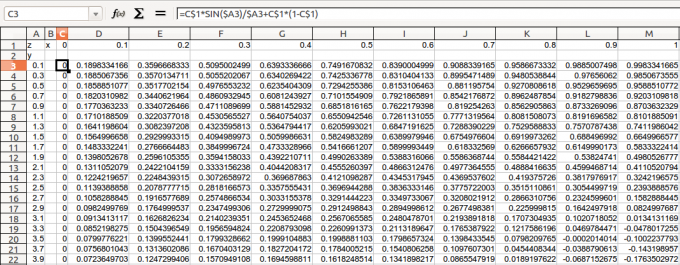UI location Usage Group: AddMesh in 3D View: Shift-A > Mesh > Data Surface 1.1 Sun Sibai, Pontiac 2.71 or later GPL v2 or later Extern Data files contain matrices of x,y,z coordinates of vertices.

## Introduction

In engineering and science, numerical data sometimes are more common than analytical expressions. Analytical expressions are accurate and easy to accept, but for numerical algorithms (such as self-adapting optimizing) and real experiments (such as measurements with instruments), numerical data are more suitable and more convenient.

If you want to transfer data from numerical algorithms or real experiments into Blender to visualize them, this addon may be your proper choice. In the example I will show below, I turned text data of x,y and z coordinates into a mesh surface.

If you are seeking for addons for analytical expressions, please go to the addon 3D Function Surface.

## Installation

Then copy directory add_mesh_DataSurface to Blender addon path. If you don't know where is the addon path, please open your Blender, and run scripts below in the Python Console:

```import addon_utils
```

• Click Files > User preferences ... > Addons > Add mesh.

## Instructions

There are two modules in this addon:

• Text Data Surface can create all mesh surface in 3D space. But it needs 3 matrices data:
```x=x(u,v)
y=y(u,v)
z=z(u,v)
```

where x,y,z are coordinates in space and u,v are column and row indices.

• Z Data Surface can create a subset mesh surface of above. It is convenient for only 1 table data needed:
```z=f(x,y)
```

where x,y data are in form of vector and z data are in form of matrix.

To show how to use this addon, I will give examples.

### example for Text Data Surface

• In the 3D View block, press Shift+a > Mesh > Data surface > Text data surface.

Then a twisted ribbon-like mesh shows.

The coordinates of vertices are from data files Xdata.txt, Ydata.txt and Zdata.txt.
These data can be generated from Octave/Matlab script example.m.
To change the data files, clicking the browsing buttons (shown right).

As for the loop and flip checkbox, try them to know what they represent. After clicking loop, you should see the figure closed in each Z slice. Yes, the ribbon becomes a tube. After clicking flip, you should see the light effect changed a little. Yes, the normal direction flipped.

### example for Z Data Surface

• In the 3D View block, press Shift+a > Mesh > Data surface > Z(x,y) table surface.

Then a floating ribbon-like mesh shows.

The coordinates of vertices are from table data file csvdata.csv.
To change the data files, clicking the browsing button (shown right).
Plain text table is also OK.

The structure of the table in .csv file is shown below:

The .csv file can be exported from spreadsheet programs such as Excel and LibreOffice Calc.There are 3 boolean options in Z Data Surface. loop and flip are like those in Text Data Surface.

Here shows their effects.

The tran option is to switch x axis with y axis. It is the same with transposing the whole data table. By combining tran function and loop function, we can close the surface mesh either in x direction or y direction, shown below: